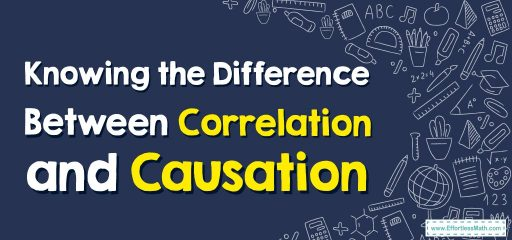# How to Know the Difference Between Correlation and CausationCorrelation and causation are two statistical concepts that, although they are related, have differences from each other.

Correlation shows the relationship between two variables.

Causation shows the relationship between an independent variable and a dependent variable. In Causation, any changes in the independent variable lead to changes in the dependent variable.

It is possible to solve the problems related to correlation and causation in the following way:

Step 1: Identify the variables and specify the type of each. Variables can be categorical or numerical.

Step 2: Gather data related to variables and organize it a table or graph in such a way that can be relevant and meaningful.

Step 3: Determine the strength and direction of the correlation between variables. For this purpose, you can find the correlation coefficient such as Pearson’s r or Spearman’s rho.

Step 4: After finding the correlation coefficient, interpret it. If the correlation coefficient is positive, it shows that the variables increase or decrease together. If the correlation coefficient is negative, it shows that there is an inverse relationship between the changes of the variables. If the correlation coefficient is zero, there isn’t any correlation between the variables.

Step 5: Try to find information that can imply a causal relationship. This information can be of the type of finding a reasonable mechanism that links the variables together or of the type of change in the independent variable before the change in the dependent variable.

Step 6: There are many statistical methods that can be used to determine the direction and strength of a causal relationship. Among these methods, regression analysis, experiment, or observational study can be mentioned.

Note: In addition to correlation, you should also look for other factors that may determine the relationship and causality because correlation does not mean causation.

### What people say about "How to Know the Difference Between Correlation and Causation - Effortless Math: We Help Students Learn to LOVE Mathematics"?

No one replied yet.

X
30% OFF

Limited time only!

Save Over 30%

SAVE $5 It was$16.99 now it is \$11.99